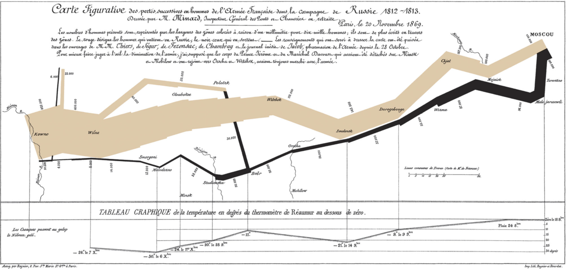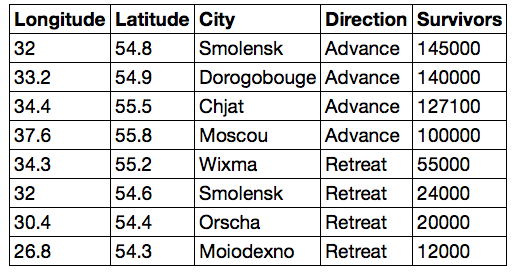# 三、Python 编程

## 表达式

``````3 * 4
12
``````

``````3 * * 4
File "<ipython-input-4-d90564f70db7>", line 1
3 * * 4
^
SyntaxError: invalid syntax
``````

``````3 ** 4
81
``````

Python 表达式遵循熟悉的优先级规则，与代数中相同：乘法和除法在加法和减法之前计算。 圆括号可以用来在较大的表达式中，将较小的表达式组合在一起。

``````1 + 2 * 3 * 4 * 5 / 6 ** 3 + 7 + 8 - 9 + 10
17.555555555555557
1 + 2 * (3 * 4 * 5 / 6) ** 3 + 7 + 8 - 9 + 10
2017.0
``````

### 示例Ross Ihaka 的图片版本

``````(25050 - 3262)/(1963 - 1939)
907.8333333333334
``````

``````(62799 - 25050)/(1976 - 1963)
2903.769230769231
``````

## 数值

### 整数值

• 整数在 Python 语言中称为`int`值。 它们只能表示没有小数部分的整数（负数，零或正数）
• 实数在 Python 语言中被称为`float`值（或浮点值）。 他们可以表示全部或部分数字，但有一些限制。

``````# Some int values
2
2
1 + 3
4
-1234567890000000000
-1234567890000000000
# Some float values
1.2
1.2
1.5 + 2
3.5
3 / 1
3.0
-12345678900000000000.0
-1.23456789e+19
``````

### 浮点值

`float`可以表示非常大和非常小的数字。存在限制，但你很少遇到他们。 浮点数只能表示任何数字的 15 或 16 位有效数字；剩下的精度就会丢失。 这个有限的精度对于绝大多数应用来说已经足够了。 将浮点值与算术运算结合后，最后的几位数字可能不正确。 第一次遇到时，微小的舍入错误往往令人困惑。

``````2e306 * 10
2e+307
2e306 * 100
inf
2e-322 / 10
2e-323
2e-322 / 100
0.0
``````

``````0.6666666666666666 - 0.6666666666666666123456789
0.0
``````

``````2 ** 0.5
1.4142135623730951
(2 ** 0.5) * (2 ** 0.5)
2.0000000000000004
(2 ** 0.5) * (2 ** 0.5) - 2
4.440892098500626e-16
``````

## 名称

``````a = 10
b = 20
a + b
30
``````

``````quarter = 1/4
half = 2 * quarter
half
0.5
``````

``````quarter = 4
half
0.5
``````

``````purchase_price = 5
state_tax_rate = 0.075
county_tax_rate = 0.02
city_tax_rate = 0
sales_tax_rate = state_tax_rate + county_tax_rate + city_tax_rate
sales_tax = purchase_price * sales_tax_rate
sales_tax
0.475
``````

## 示例：增长率

``````initial = 2766000
changed = 2814000
(changed - initial) / initial
0.01735357917570499
``````

``````(changed/initial) - 1
0.017353579175704903
``````

``````initial = 2766
changed = 2814
(changed/initial) - 1
0.017353579175704903
``````

10 年以来，美国联邦政府的雇员人数仅增长了 1.74%。 那个时候，美国联邦政府的总支出从 2.37 万亿美元增加到 2012 年的 3.38 万亿美元。

``````initial = 2.37
changed = 3.38
(changed/initial) - 1
0.4261603375527425
``````

``````initial = 287.6
changed = 314.1
(changed/initial) - 1
0.09214186369958277
``````

``````initial = 15.3
changed = 11.9
(changed/initial) - 1
-0.2222222222222222
``````

``````1.035 * 1.035 * 1.035 * 1.035 * 1.035 * 1.035 * 1.035 * 1.035 * 1.035 * 1.035 - 1
0.410598760621121
``````

``````annual_growth_rate = 0.035
ten_year_growth_rate = (1 + annual_growth_rate) ** 10 - 1
ten_year_growth_rate
0.410598760621121
``````

``````initial = 2.37
changed = 3.38
t = 10
(changed/initial) ** (1/t) - 1
0.03613617208346853
``````

``````initial * (1 + g) ** t
``````

``````(changed/initial) ** (1/t) - 1
``````

## 调用表达式

``````abs(-12)
12
round(5 - 1.3)
4
max(2, 2 + 3, 4)
5
``````

``````import math
import operator
3.0
``````

``````(4 + 5) ** 0.5
3.0
``````

``````florida = 2.72
iowa = 16.25
100*abs(florida-iowa)/((florida+iowa)/2)
142.6462836056932
``````

``````math.log?
log(x[, base])

Return the logarithm of x to the given base.
If the base not specified, returns the natural logarithm (base e) of x.
``````

``````math.log(16, 2)
4.0
math.log(16)/math.log(2)
4.0
``````

Python 的内建函数列表非常长，包含了许多在数据科学应用中不需要的函数。 `math`模块中的数学函数列表同样很长。 本文将在上下文中介绍最重要的函数，而不是期望读者记住或理解这些列表。

### 示例

1869 年，一位名叫查尔斯·约瑟夫·米纳德（Charles Joseph Minard）的法国土木工程师，创造了一个图表，仍被认为是有史以来最伟大的图表之一。 它显示了拿破仑军队从莫斯科撤退期间的损失。 1812 年，拿破仑开始征服俄罗斯，他的军队中有超过 35 万人。 他们确实到达了莫斯科，但是沿路一直受到损失的困扰。 俄国军队不断撤退到俄罗斯深处，故意焚烧田野，并在撤退时摧毁村庄。 这使法国军队在俄罗斯冬季来临之时，没有食物或避难所。法国军队在莫斯科没有取得决定性的胜利就撤退了。 之后天气变冷，死了更多的人。 回来的人还不到一万。Minard 的地图

• 士兵的数量
• 行军的方向
• 位置的经纬度
• 回程的温度
• 十一月和十二月的具体日期的位置

Tufte 说 Minard 的图是“可能是有史以来最好的统计图表”。Minard 的子集

``````moscou = 100000
wixma = 55000
wixma - moscou
-45000
(wixma - moscou)/moscou
-0.45
``````

``````smolensk_A = 145000
moiodexno = 12000
(moiodexno - smolensk_A)/smolensk_A
-0.9172413793103448
``````

``````dorogobouge = 140000
smolensk_R = 24000
orscha = 20000
abs(dorogobouge - smolensk_A)
5000
abs(dorogobouge - smolensk_A)/smolensk_A
0.034482758620689655
abs(orscha - smolensk_R)
4000
abs(orscha - smolensk_R)/smolensk_R
0.16666666666666666
``````